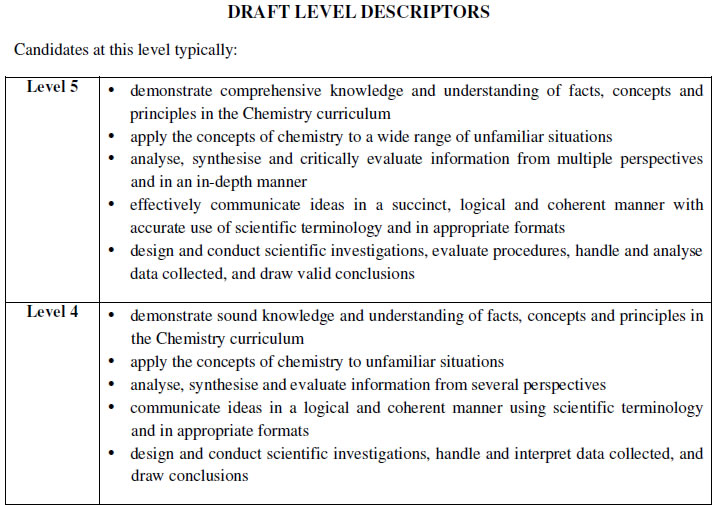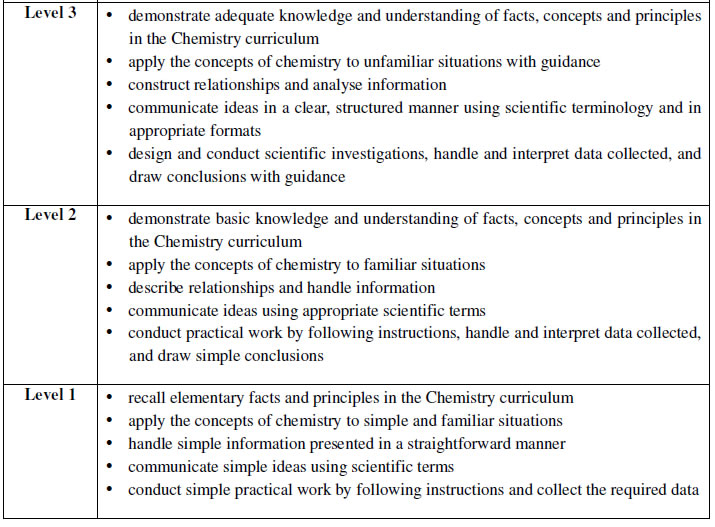HKDSE Chemistry
IGCSE Chemistry
IAL Chemistry
GCE Chemistry
IB Chemistry
tutorial

Gary Sir

* HKU Chemistry
* HKCEE Chemistry A
* HKALE Chemistry A
* HKDSE Chemistry 5** x 2

DSE Chemistry tutor
IAL Chemistry tutor
GCE Chemistry tutor

Contact gary
60551219 (whatsapp)Enquiry:
gary@chemistryhk.com

# HKDSE Chemistry化學 私人補習 Chemistry tutorial

### 距離2020 DSE Chemistry 考試尚餘: //################################################################### // Author: ricocheting.com // Version: v3.1 // Date: 2017-01-03 // Description: displays the amount of time until the "dateFuture" entered below. var CDown = function() { this.state=0;// if initialized this.counts=[];// array holding countdown date objects and id to print to {d:new Date(2013,11,18,18,54,36), id:"countbox1"} this.interval=null;// setInterval object } CDown.prototype = { init: function(){ this.state=1; var self=this; this.interval=window.setInterval(function(){self.tick();}, 1000); }, add: function(date,id){ this.counts.push({d:date,id:id}); this.tick(); if(this.state==0) this.init(); }, expire: function(idxs){ for(var x in idxs) { this.display(this.counts[idxs[x]], "Now!"); this.counts.splice(idxs[x], 1); } }, format: function(r){ var pre='',post='',divide=', ', out=""; if(r.d != 0){out += pre+r.d +" "+((r.d==1)?"day":"days")+post+divide;} return out.substr(0,out.length-divide.length); }, math: function(work){ var y=w=d=h=m=s=ms=0; ms=(""+((work%1000)+1000)).substr(1,3); work=Math.floor(work/1000);//kill the "milliseconds" so just secs y=Math.floor(work/31536000);//years (no leapyear support) w=Math.floor(work/604800);//weeks d=Math.floor(work/86400);//days work=work%86400; h=Math.floor(work/3600);//hours m=Math.floor(work/60);//minutes s=Math.floor(work);//seconds return {y:y,w:w,d:d,h:h,m:m,s:s,ms:ms}; }, tick: function(){ var now=(new Date()).getTime(), expired=[],cnt=0,amount=0; if(this.counts) for(var idx=0,n=this.counts.length; idx<n; ++idx){ cnt=this.counts[idx]; amount=cnt.d.getTime()-now;//calc milliseconds between dates // if time is already past if(amount<0){ expired.push(idx); } // date is still good else{ this.display(cnt, this.format(this.math(amount))); } } // deal with any expired if(expired.length>0) this.expire(expired); // if no active counts, stop updating if(this.counts.length==0) window.clearTimeout(this.interval); }, display: function(cnt,msg){ document.getElementById(cnt.id).innerHTML=msg; } }; window.onload=function(){ var cdown = new CDown(); cdown.add(new Date(2020,2,28,8,30,0), "countbox1"); };

DSE Chem course content:
Topic I. Planet earth
Topic II. Microscopic world I
Topic III. Metals
Topic IV. Acids and bases
Topic V. Fossil fuels and carbon compounds
Topic VI. Microscopic world II
Topic VII. Redox reactions, chemical cells and electrolysis
Topic VIII. Chemical reactions and energy
Topic IX. Rate of reaction
Topic X. Chemical equilibrium
Topic XI. Chemistry of carbon compounds
Topic XII. Patterns in the chemical world
Topic XIII. Industrial chemistry
Topic XIV. Material chemistry
Topic XV. Analytical chemistry

 Component Weighting Duration Paper 1: Compulsory part of the curriculum 60% 2 hours 30 minutes Paper 2: Elective part of the curriculum 20% 1 hour School-based Assessment (SBA) 20%

Paper 1 comprises two sections: A and B.

• Section A consists of multiple-choice questions and carries 18% of the subject mark.
• Section B includes short questions, structured questions and essay questions, and carries 42% of the subject mark.
• In each of the sections A and B, Part I will set questions mainly on topics I to VIII of the curriculum, while Part II mainly on topics IX to XII.
• Candidates have to attempt all questions in this paper.

Paper 2 consists of structured questions and carries 20% of the subject mark.

• Candidates are required to answer the questions on the 2 electives selected